• 产生一组 mxn 的正交向量， 输入应该是两个标量，m 和 n，其中 n 小于或等于 m。 示例：>> get_orthonormal(5,4) 答案 = 0.1503 -0.0884 -0.0530 0.8839 -0.4370 -0.7322 -0.1961 -0.2207 -0.3539 0.3098 0.7467 ...matlab
• ## 正交向量 正交矩阵

千次阅读 2017-11-12 22:36:10
正交向量 正交矩阵
正交向量
“正交向量”是一个数学术语，指点积为零的两个或多个向量
换句话说， 两个向量正交意味着它们是相互垂直的。若向量α与β正交，则记为α⊥β

如何求解正交矩阵：
https://jingyan.baidu.com/article/9faa72318364a7473c28cbe9.html

正交矩阵

正交矩阵是实数特殊化的酉矩阵，因此总是正规矩阵。尽管我们在这里只考虑实数矩阵，这个定义可用于其元素来自任何域的矩阵。正交矩阵毕竟是从内积自然引出的，对于复数的矩阵这导致了归一要求。

定义
定义 1

如果：AA'=E（E为单位矩阵，A'表示“矩阵A的转置矩阵”。）或A′A=E，则n阶实矩阵 A称为正交矩阵， 若A为正交阵，则满足以下条件:

1) A 是正交矩阵

3) A′是正交矩阵

4) A的各行是单位向量且两两正交

5) A的各列是单位向量且两两正交

6) (Ax,Ay)=(x,y) x,y∈R

正交矩阵通常用字母Q表示。

举例：A=[r11 r12 r13;r21 r22 r23;r31 r32 r33]

则有：
r11^2+r12^2+r13^2=r21^2+r22^2+r23^2=r31^2+r32^2+r33^2=1

r11*r12+r21*r22+r31*r32=0等性质

正交方阵是欧氏空间中标准正交基到标准正交基的过渡矩阵。

在矩阵论中，实数正交矩阵是方块矩阵 Q，它的转置矩阵是它的逆矩阵:

，如果正交矩阵的行列式为 +1，则我们称之为特殊正交矩阵:

概述
要看出与内积的联系，考虑在 n 维实数内积空间中的关于正交基写出的向量v。v 的长度的平方是vv。如果矩阵形式为Qv 的线性变换保持了向量长度，则

所以有限维线性等距同构，比如旋转、反射和它们的组合，都产生正交矩阵。反过来也成立:
正交矩阵蕴涵了正交变换。但是，线性代数包括了在既不是有限维的也不是同样维度的空间之间的正交变换，它们没有等价的正交矩阵。

有多种原由使正交矩阵对理论和实践是重要的。n×n 正交矩阵形成了一个群，即指示为O(n) 的正交群，它和它的子群广泛的用在数学和物理科学中。例如，分子的点群是O(3)
的子群。因为浮点版本的正交矩阵有有利的性质，它们是字数值线性代数中很多算法比如 QR分解的关键，通过适当的规范化，离散余弦变换 (用于MP3 压缩)可用正交矩阵表示。

例子
下面是一些小正交矩阵的例子和可能的解释。

恒等变换。 旋转 16.26°。 针对 x 轴反射。 旋转反演(rotoinversion): 轴 (0,-3/5,4/5)，角度90°。 置换坐标轴。

基本构造

低维度
最简单的正交矩阵是 1×1 矩阵  和 [−1]，它们可分别解释为恒等和实数线针对原点的反射。

如下形式的 2×2 矩阵

它的正交性要求满足三个方程

在考虑第一个方程时，不丢失一般性而设 p = cos θ, q = sin θ；因此要么 t = −q,u =p 要么t = q, u = −p。我们可以解释第一种情况为旋转 θ(θ = 0 是单位矩阵)，第二个解释为针对在角 θ/2 的直线的反射。

旋转 反射 在 45°的反射对换 x 和 y；它是置换矩阵，在每列和每行带有一个单一的 1(其他都是 0):

单位矩阵也是置换矩阵。

反射是它自己的逆，这蕴涵了反射矩阵是对称的(等于它的转置矩阵)也是正交的。两个旋转矩阵的积是一个旋转矩阵，两个反射矩阵的积也是旋转矩阵。

更高维度
不管维度，总是可能把正交矩阵按纯旋转与否来分类，但是对于 3×3 矩阵和更高维度矩阵要比反射复杂多了。例如，

和 表示通过原点的反演和关于z 轴的旋转反演(逆时针旋转90°后针对x-y平面反射，或逆时针旋转 270°后对原点反演)。

旋转也变得更加复杂；它们不再由一个角来刻画，并可能影响多于一个平面子空间。尽管经常以一个轴和角来描述 3×3 旋转矩阵，在这个维度旋转轴的存在是偶然的性质而不适用于其他维度。

但是，我们有了一般适用的基本建造板块如置换、反射、和旋转。

基本变换
最基本的置换是换位(transposition)，通过交换单位矩阵的两行得到。任何 n×n 置换矩阵都可以构造为最多 n−1 次换位的积。 构造自非零向量 v 的 Householder反射为

这里的分子是对称矩阵，而分母是 v 的平方量的一个数。这是在垂直于 v 的超平面上的反射(取负平行于v 任何向量分量)。如果v 是单位向量，则Q = I−2vv 就足够了。Householder 反射典型的用于同时置零一列的较低部分。任何n×n 正交矩阵都可以构造为最多n 次这种反射的积。

Givens旋转作用于由两个坐标轴所生成的二维(平面)子空间上，按选定角度旋转。它典型的用来置零一个单一的次对角线元素(subdiagonal entry)。任何n×n 的旋转矩阵都可以构造为最多n(n−1)/2 次这种旋转的积。在 3x3 矩阵的情况下，三个这种旋转就足够了；并且通过固定这个序列，我们可以用经常叫做欧拉角的三个角来(尽管不唯一)描述所有
3×3 旋转矩阵。

雅可比旋转有同 Givens 旋转一样的形式，但是被用做相似变换，选择来置零 2×2 子矩阵的两个远离对角元素(off-diagonal entry)。

性质

矩阵性质
实数方块矩阵是正交的，当且仅当它的列形成了带有普通欧几里得点积的欧几里得空间R 的正交规范基，它为真当且仅当它的行形成R 的正交基。假设带有正交(非正交规范)列的矩阵叫正交矩阵可能是诱人的，但是这种矩阵没有特殊价值而没有特殊名字；他们只是MM =D，D 是对角矩阵。

任何正交矩阵的行列式是 +1 或 −1。这可从关于行列式的如下基本事实得出:

反过来不是真的；有 +1 行列式不保证正交性，即使带有正交列，可由下列反例证实。

对于置换矩阵，行列式是 +1 还是 −1 匹配置换是偶还是奇的标志，行列式是行的交替函数。

比行列式限制更强的是正交矩阵总可以是在复数上可对角化来展示特征值的完全的集合，它们全都必须有(复数)绝对值 1。

群性质
正交矩阵的逆是正交的，两个正交矩阵的积是正交的。事实上，所有 n×n 正交矩阵的集合满足群的所有公理。它是 n(n−1)/2 维的紧致李群，叫做正交群并指示为O(n)。

行列式为 +1 的正交矩阵形成了路径连通的子群指标为 2 的 O(n) 正规子群，叫做旋转的特殊正交群 SO(n)。商群O(n)/SO(n)
同构于O(1)，带有依据行列式选择 [+1] 或 [−1] 的投影映射。带有行列式 −1 的正交矩阵不包括单位矩阵，所以不形成子群而只是陪集；它也是(分离的)连通的。所以每个正交群被分为两个部分；因为投影映射分裂，O(n)
是 SO(n) 与 O(1)的半直积。用实用术语说，一个相当的陈述是任何正交矩阵可以通过采用一个旋转矩阵并可能取负它的一列来生成，如我们在 2×2 矩阵中看到的。如果n 是奇数，则半直积实际上是直积，任何正交矩阵可以通过采用一个旋转矩阵并可能取负它的所有列来生成。

现在考虑 (n+1)×(n+1) 右底元素等于 1 的正交矩阵。最后一列(和最后一行)的余下元素必须是零，而任何两个这种矩阵的积有同样的形式。余下的矩阵是n×n 正交矩阵；因此O(n) 是O(n+1) (和所有更高维群)的子群。

因为 Householder 正交矩阵形式的基本反射可把任何正交矩阵简约成这种约束形式，一系列的这种反射可以把任何正交矩阵变回单位矩阵；因此正交群是反射群。最后一列可以被固定为任何单位向量，并且每种选择给出不同的O(n) 在O(n+1) 中的复本；以这种方式O(n+1) 是在单位球S 与纤维 O(n) 上的丛。

类似的，SO(n) 是 SO(n+1) 的子群；任何特定正交矩阵可以使用类似过程通过 Givens 平面旋转来生成。丛结构持续:SO(n) ↪SO(n+1) →S。一个单一旋转可以在最后一列的第一行生成一个零，而n−1 次旋转序列将置零n×n 旋转矩阵的除了最后一列的最后一行的所有元素。因为平面是固定的，每次旋转只有一个自由度，就是它的角度。通过归纳，SO(n)
因此有

自由度，O(n) 也是。

置换矩阵简单一些；它们不形成李群，只是一个有限群，n! 次对称群Sn。通过同类的讨论，Sn 是Sn+1 的子群。偶置换生成行列式 +1 的置换矩阵的子群，n!/2
次交错群。

规范形式
更广泛的说，任何正交矩阵的效果分离到在正交二维空间上的独立动作。就是说，如果 Q 是狭义正交的，则你可以找到(旋转)改变基的一个正交矩阵 P，把 Q 带回到分块对角形式:

(n 偶数)， (n 奇数)。 这里的矩阵 R1,...,Rk 是 2×2 旋转矩阵，而余下的元素是零。作为例外，一个旋转块可以是对角的， ±I。因此如果需要的话取负一列，并注意 2×2 反射可对角化为 +1 和 −1，任何正交矩阵可变为如下形式

, 矩阵 R1,…,Rk 给出位于复平面中单位圆上的特征值的共轭对；所以这个分解复合确定所有带有绝对值 1 的特征值。如果n 是奇数，至少有一个实数特征值 +1 或 −1；对于 3×3
旋转，关联着 +1 的特征向量是旋转轴。

数值线性代数

利益
数值分析自然的利用了正交矩阵的很多数值线性代数的性质。例如，经常需要计算空间的正交基，或基的正交变更；二者都采用了正交矩阵的形式。有行列式 ±1 和所有模为 1 的特征值是对数值稳定性非常有利的。 一个蕴涵是条件数为
1 (这是极小的)，所以在乘以正交矩阵的时候错误不放大。很多算法为此使用正交矩阵如 Householder反射和 Givens旋转。有帮助的不只是正交矩阵是可逆的，还有它的逆矩阵本质上是免花费的，只需要对换索引(下标)。

置换是很多算法成功的根本，包括有局部定支点(partial pivoting)的运算繁重的高斯消去法(这里的置换用来定支点)。但是它们很少明显作为矩阵出现；它们的特殊形式允许更有限的表示，比如n 个索引的列表。

同样的，使用 Householder 和 Givens 矩阵的算法典型的使用特殊方法的乘法和存储。例如，Givens 旋转只影响它所乘的矩阵的两行，替代完全的n 次的矩阵乘法为更有效的n 次运算。在使用这些反射和旋转向矩阵介入零的时候，腾出的空间足够存储充足的数据来重生成这个变换。

分解
一些重要的矩阵分解(Golub & Van Loan, 1996)涉及到了正交矩阵，包括:

QR分解 M = QR, Q 正交，R 上三角。 奇异值分解 M = UΣV, U 和 V 正交，Σ 非负对角。
谱分解S =QΛQ,S 对称，Q 正交，Λ 对角。 极分解 M =QS,Q 正交，S 对称非负确定。


展开全文• 正交向量与正交化线性相关正交向量基正交化 线性相关 定义 定义1：在向量空间 VVV 的一组向量 AAA ：α1,α2,⋯αm\alpha_{1},\alpha_{2},\cdots \alpha_{m}α1​,α2​,⋯αm​，如果存在不全为零的数 k1,k2,⋯...
正交向量与正交化线性相关正交向量正交基正交化
线性相关

定义

定义1：在向量空间 $V$ 的一组向量 $A$ ：$\alpha_{1},\alpha_{2},\cdots \alpha_{m}$，如果存在不全为零的数 $k_{1},k_{2},\cdots k_{m}$ ，使 $k_{1}\alpha_{1}+k_{2}\alpha_{2}+\cdots +k_{m}\alpha_{m}=0$ 则称向量组  $A$ 是线性相关的，否则，称其为线性无关的。
定义2：当且仅当 $\alpha_{1},\alpha_{2},\cdots \alpha_{m}$ 全为零时，使得使 $k_{1}\alpha_{1}+k_{2}\alpha_{2}+\cdots +k_{m}\alpha_{m}=0$  成立，称向量组线性无关。

定理：

两个向量 $a$ 、$b$ 共线的充要条件是 $a$、$b$ 线性相关；
三个向量 $a$ 、$b$ 、$c$ 共面的充要条件是 $a$ 、$b$ 、$c$ 线性相关；
向量 $a_1,a_2, ···,a_n (n\geqslant 2)$ 线性相关的充要条件是这 $n$ 个向量中的一个为其余 $(n-1)$ 个向量的线性组合，即有 $a_n$ ，且存在数域K中一组数 $k_{1},k_{2},\cdots k_{m}$ ，满足 $a_n = k_1a_1+k_2a_2 + \cdots +k_{n-1}a_{n-1}$

注意：

对于任一向量组而言,，不是线性无关的就是线性相关的；
包含零向量的任何向量组是线性相关的；
含有相互平行的向量的向量组必线性相关；
向量组是线性相关的，那么增加向量的个数，不改变向量的相关性。【局部相关，整体相关】
向量组是线性无关的，那么减少向量的个数，不改变向量的无关性。【整体无关，局部无关】

正交向量
通常，两个向量垂直的充分必要条件是它们夹角的余弦为零，亦即它们的数量积为零。在一般的欧式空间中，仍以内积定义两向量夹角的余弦。

定义：如果对于欧式空间中的两个向量 $x$ 与 $y$ 有 $(x,y)=0$ ，则称 $x$ 与 $y$ 正交 或 垂直 ，记为 $x\perp y$ 。

结论：

当  $x$ 与 $y$ 正交时， $y$ 与 $x$ 也正交；
零向量与任意向量均正交；
如果 $x\perp y$ ，且  $x$ 与 $y$ 线性相关，则此二向量中至少有一个是零向量。

定义：如果欧式空间中一组非零向量两两正交，则称为正交向量组。
定理：设 $x_{1},x_{2},\cdots x_{m}$ 是正交向量组，则它们必线性无关。

上述定理可以表明，在 $n$ 维欧式空间中，两两正交的非零向量不能超过 $n$ 个。例如，在平面上找不到三个两两正交的非零向量；在通常的三维空间 $R^3$ 中，找不到四个两两正交的非零向量。
正交基

定义：在欧式空间 $V^n$ 中，由 $n$ 个非零向量组成的正交向量组称为  $V^n$ 的 正交基 ；由单位向量组成的正交基称为 标准正交基 或 法正交基 ；其基向量称为 单位坐标向量 。

一个基为标准正交基的 充要条件 是它的度量矩阵为单位矩阵。
证明：设 $x_{1},x_{2},\cdots x_{n}$ 为标准正交基，则由定义有 $\left ( x_{i},x_{j} \right )=x_{i}^{T}x_{j}=\begin{cases} 1 & \text{ if } i=j \\ 0 & \text{ if } i\neq j \end{cases}$ 可见 ，它的度量矩阵是单位矩阵。反之，如果以单位矩阵为度量矩阵，则由矩阵相等可得 $\left ( x_{i},x_{j} \right )=x_{i}^{T}x_{j}$ ，即  $x_{1},x_{2},\cdots x_{n}$ 为标准正交基。
正交化

定理：对于欧式空间  $V^n$ 的任一基 $x_{1},x_{2},\cdots x_{n}$ ，都可以找到一个标准正交基  $y_{1},y_{2},\cdots y_{n}$ 。换言之，任一非零欧式空间都有正交基和标准正交基。

这个正交基我们可以通过Gram-Schmidt正交化获得。
Gram-Schmidt正交化方法是将 线性无关 的向量转化为 标准正交化向量 的方法。注意这里的前提，Gram-Schmidt正交化方法是对线性无关的向量操作。

假设欧式空间 $V^n$ 中的任一基为 $x_{1},x_{2},\cdots x_{n}$ ，我们通过Gram-Schmidt正交化方法得到该基的正交基和标准正交基。

取 $\beta_{1}=x_1$ ，作所求正交基中的第一个向量。
令 $\beta_{2}=x_2+k\beta_{1}$ ，由于我们要构造的正交基两两向量相互正交，有  $(\beta_{1},\beta_{2})=0$ ，通过这个条件来计算待定常数 $k$ 。
由 $(\beta_{1},x_2+k\beta_{1})=(\beta_{1},x_2)+k(\beta_{1},\beta_{1})=0$ 得 $k=-\frac{(x_2,\beta _{1})}{(\beta _{1},\beta _{1})}$ 代入 $\beta_{2}=x_2+k\beta_{1}$ ，这样就得到两个相互正交的向量 $\beta_{1}$ ，$\beta_{2}$，且 $\beta_{2} \neq 0$ 。
令 $\beta_{3}=x_3+k_2\beta_{2}+k_1\beta_{1}$ ，再由正交条件 $(\beta_{1},\beta_{3})=0$ 及$(\beta_{2},\beta_{3})=0$ 来决定出常数 $k_1$ 和 $k_2$ 为 $k_2=-\frac{(x_3,\beta _{2})}{(\beta _{2},\beta _{2})},k_1=-\frac{(x_3,\beta _{1})}{(\beta _{1},\beta _{1})}$
以此类推，继续进行下去，直到最后一个向量。

上述获得的向量组 $(\beta _{1},\beta _{2},\cdots ,\beta _{n})$ 即为欧式空间 $V^n$ 中的一个 正交基 。再将各向量单位化，即除以各自的模，得到单位正交向量组  $y_{1},y_{2},\cdots y_{n}$，也即 标准正交基。
$y_{i}=\frac{\beta_i}{\left | \beta _{i} \right |},i=1,2,...,n$ 由基  $x_{1},x_{2},\cdots x_{n}$ 求标准正交基  $y_{1},y_{2},\cdots y_{n}$ 的过程也称把基  $x_{1},x_{2},\cdots x_{n}$ 正交单位化 或 正交规范化 。
为简单起见，以包含四个向量的向量组为例，介绍Schmidt正交化的具体过程。假设向量组为 $x_{1}=\left ( 1,1,0,0 \right )$ ， $x_{2}=\left ( 1,0,1,0 \right )$ ， $x_{3}=\left ( -1,0,0,1 \right )$ ， $x_{4}=\left ( 1,-1,-1,1 \right )$ ，求解其标准正交基。
解：首先先把它们正交化。
取 $\beta_{1}=x_1=\left ( 1,1,0,0 \right )$
令 $\beta_{2}=x_2+k\beta_{1}$ ，常数 $k$ 为 $k=-\frac{(x_2,\beta _{1})}{(\beta _{1},\beta _{1})}=-\frac{1}{2}$，则
\begin{aligned} \beta_{2}&=x_2+k\beta_{1} \\ &=\left ( 1,0,1,0 \right )-\frac{1}{2}\left ( 1,1,0,0 \right )\\ &=(\frac{1}{2},-\frac{1}{2},1,0) \end{aligned}
令 $\beta_{3}=x_3+k_2\beta_{2}+k_1\beta_{1}$ ，常数 $k_1$ 与 $k_2$ 为 $k_1=-\frac{(x_3,\beta _{1})}{(\beta _{1},\beta _{1})}=\frac{1}{2},k_2=-\frac{(x_3,\beta _{2})}{(\beta _{2},\beta _{2})}=-\frac{-1/2}{3/2}=\frac{1}{3}$， 则
\begin{aligned} \beta_{3} &= x_3+k_2\beta_{2}+k_1\beta_{1}\\ &= \left ( -1,0,0,1 \right )+\frac{1}{3}(\frac{1}{2},-\frac{1}{2},1,0)+\frac{1}{2}\left ( 1,1,0,0 \right )\\ &= (-\frac{1}{3},\frac{1}{3},\frac{1}{3},1) \end{aligned}
令 $\beta_{4}=x_4+k_3\beta_{3}+k_2\beta_{2}+k_1\beta_{1}$ ，常数 $k_1$ 与 $k_2$ 为 $k_1=-\frac{(x_4,\beta _{1})}{(\beta _{1},\beta _{1})}=0,k_2=-\frac{(x_4,\beta _{2})}{(\beta _{2},\beta _{2})}=0，k_3=-\frac{(x_4,\beta _{3})}{(\beta _{3},\beta _{3})}=0$， 则 $\beta_{4}=x_4+k_3\beta_{3}+k_2\beta_{2}+k_1\beta_{1}= ( 1,-1,-1,1 )$
再单位化，便有
$y_{1}=\frac{\beta_1}{\left | \beta _{1} \right |}=\frac{1}{\sqrt{2}}\beta _{1}=(\frac{1}{\sqrt{2}},\frac{1}{\sqrt{2}},0,0)$ $y_{2}=\frac{\beta_2}{\left | \beta _{2} \right |}=\frac{2}{\sqrt{6}}\beta _{2}=(\frac{1}{\sqrt{6}},-\frac{1}{\sqrt{6}},\frac{2}{\sqrt{6}},0)$ $y_{3}=\frac{\beta_3}{\left | \beta _{3} \right |}=\frac{\sqrt{3}}{2}\beta _{3}=(-\frac{1}{\sqrt{12}},\frac{1}{\sqrt{12}},\frac{1}{\sqrt{12}},\frac{3}{\sqrt{12}})$  $y_{4}=\frac{\beta_4}{\left | \beta _{4} \right |}=\frac{1}{2}\beta _{4}=(\frac{1}{2},-\frac{1}{2},-\frac{1}{2},\frac{1}{2})$


展开全文线性代数 矩阵
• 024 正交向量 正交矩阵及性质
024 正交向量 正交矩阵及性质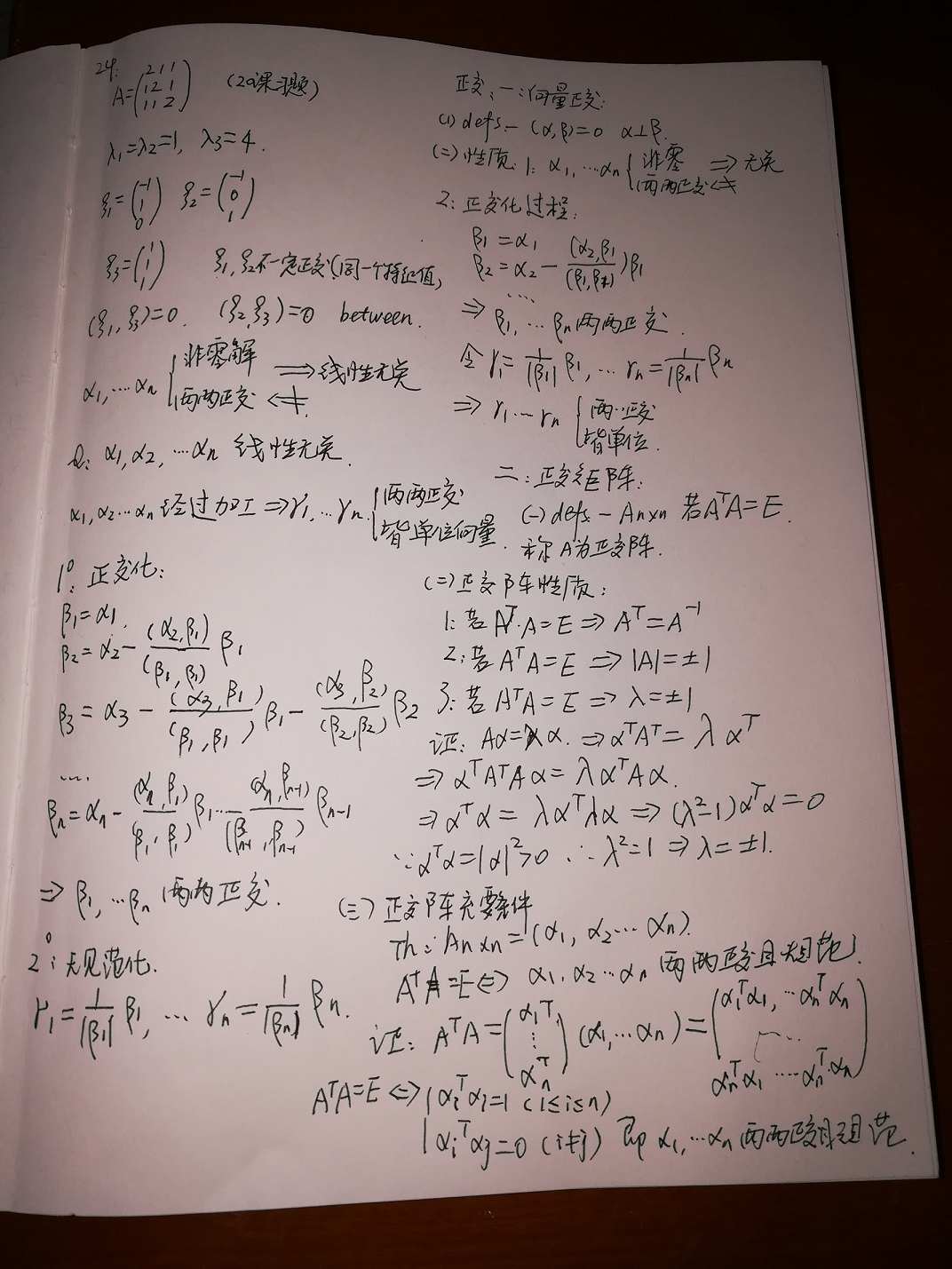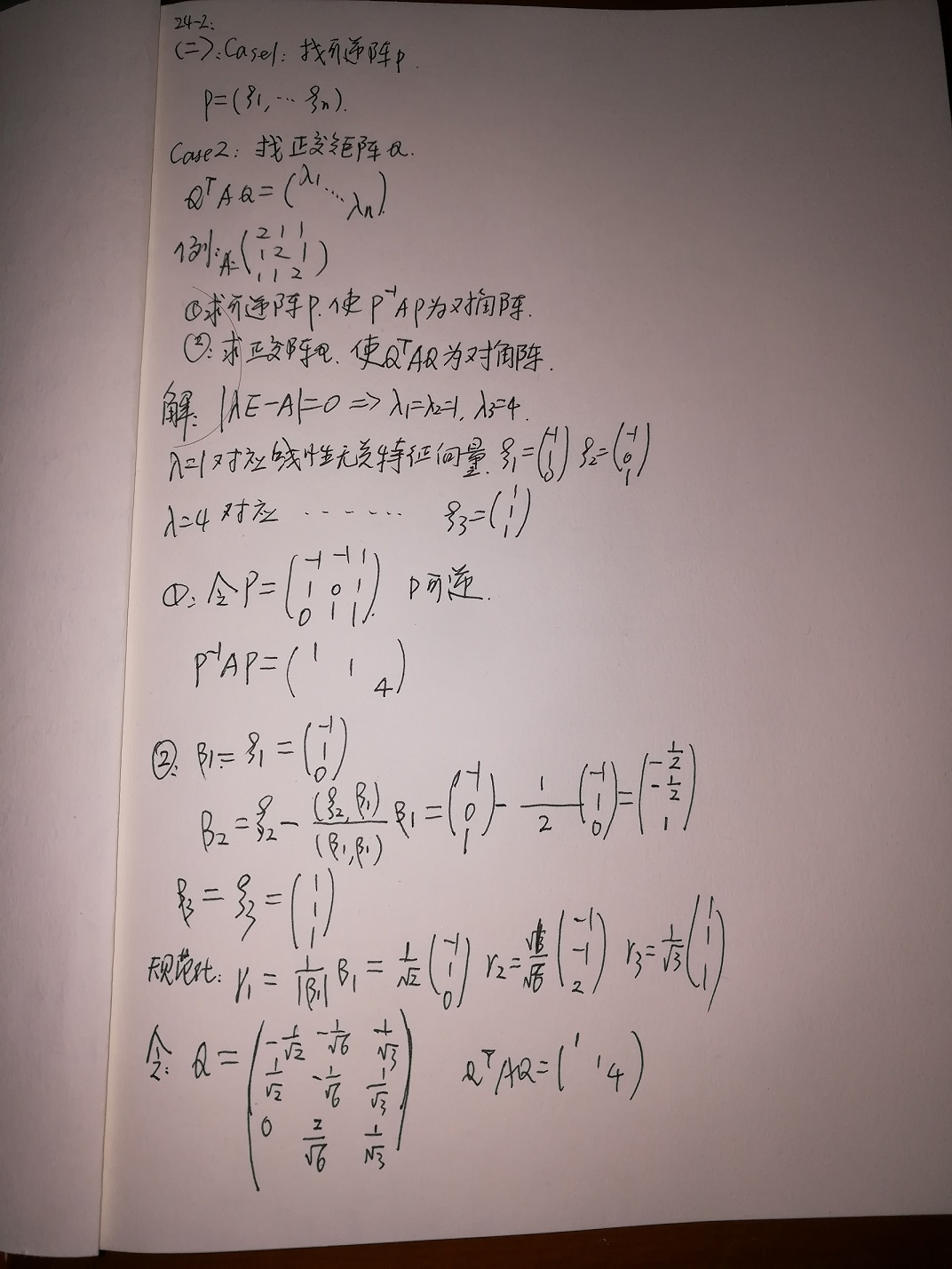展开全文矩阵 矩阵对角化
• 在前面文章《矩阵的四个基本子空间》中提到：  一个秩为r，m*n的矩阵A中，其行空间和列空间的维数为r，零空间和左零空间的维数分别为n-r，m-r，并且有...我们首先从我们熟悉的正交向量说起。 1、正交向量
在前面文章《矩阵的四个基本子空间》中提到：
一个秩为r，m*n的矩阵A中，其行空间和列空间的维数为r，零空间和左零空间的维数分别为n-r，m-r，并且有行空间与零空间正交，列空间与左零空间正交。
“掌握上面的这个结论就掌握了线性代数的半壁江山！”，MIT教授如是说。那么什么是正交子空间呢？我们首先从我们熟悉的正交向量说起。

1、正交向量
我们都知道，如果两个向量x,y正交，则其夹角为90度，可表示为表达式：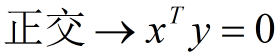注意：x，y的顺序没有区别，即下式也成立：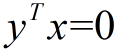两个向量正交，可以表示为下图：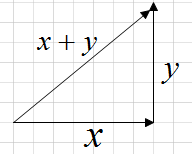由勾股定理可知：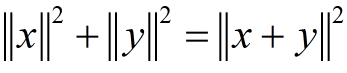将上式展开得：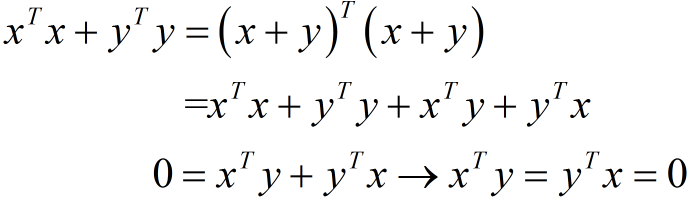我们举例说明：假设两个向量分别为x,y，z=x+y：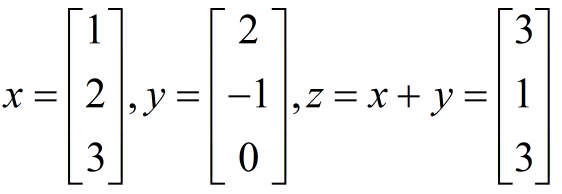其中x，y满足下式：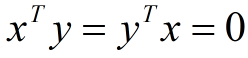则向量的长度（即向量的2范数）为：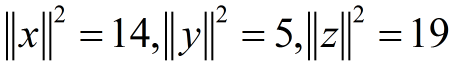显然满足勾股定理：

上面的推导，已证明勾股定理，自己可以仔细领会。

2、正交子空间
定义：两个子空间正交即两个子空间的任意两个向量正交。
文章开头说到，行空间与零空间正交，列空间与左零空间正交。下面我们来证明行空间与零空间正交，列空间与左零空间正交。

行空间与零空间正交的证明
在《矩阵的零空间》一文中，我们知道，Ax=0的解就是矩阵的零空间，则：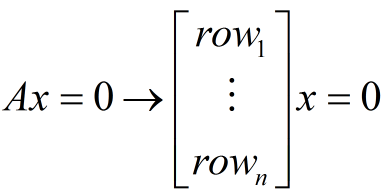展开可得：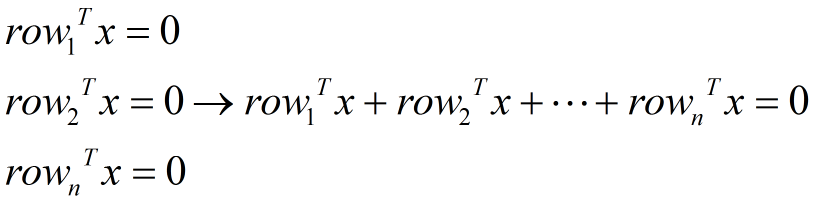上式说明，矩阵的每一行向量都与零空间正交，而矩阵的行空间就是其行向量的线性组合，则说明行空间与零空间正交。同理，我们亦可以证明列空间与左零空间正交，在此就不重复了。

原文：http://blog.csdn.net/tengweitw/article/details/41171579

展开全文线性代数 正交空间
• 利用线性代数方法研究了矩阵伸缩的高维双正交向量小波包，并给出了L2（R5）上非张量积双正交向量小波包基。
• 在前面文章《矩阵的四个基本子空间》中提到： 一个秩为r，m*n的矩阵A中，其行空间和列空间的维数为r，零空间和左零空间的维数分别为n-r，m-r，...我们首先从我们熟悉的正交向量说起。 1、正交向量 我们都知...线性代数 正交空间
• 正交向量 在我的博文程序员的自我修养之数学基础02中介绍了向量内积的概念。我们知道，对于 n维向量，其内积为： 仔细观察一下这个表达式，我们不难得出向量内积与矩阵乘法之间的联系： 回顾了向量内积之后，...正交矩阵 正交变换 标准正交基
• 正交向量 特殊的零向量 平行向量 如果一个向量是另一个向量的纯量倍数，那么这两个向量是平行向量。 请注意，v 也和零向量平行。v 也和它自己平行。 即使两个向量指向相反的方向也是平行向量。 正交向量...
• 正交向量 　正交是垂直的令一种说法，两个向量正交意味着两个向量的夹角是90°。 　这可以用直角三角形的三边解释： 　当x和y正交时，二者的点积是0，反过来也一样。这个结论在n维空间也适用，当Rn空间内的两个...正交子空间 正交补
• 1. 正交向量组的定义 2. 正交的判定 3. 正交向量组必然线性无关，线性无关向量组未必是正交向量组 4. 正交向量组求解示例 5. 标准正交向量组的定义 ...
• 文章研究了一类多元双正交向量值小波包的构造及其性质。
• 第十四课时：正交向量与子空间 本文讲解什么是向量的正交，什么是子空间的正交，什么是基的正交。 正交向量 在n维空间中，向量之间的夹角是90度 判断两个向量X,YX,YX,Y是否正交，求乘积XTYXTYX^...线性代数
• 正交向量 编辑 本词条由“科普中国”百科科学词条编写与应用工作项目审核。 “正交向量”是一个数学术语，指点积为零的两个或多个向量。几何向量的概念在线性代数中经由抽象化，得到更一般的向量概念。此处...
• 正交向量 引出 1.毕达哥拉斯定理/勾股定理（Pythagoras） 我们很容易得出 ∣∣x∣∣+∣∣y∣∣=∣∣x+y∣∣||x||+||y||=||x+y||∣∣x∣∣+∣∣y∣∣=∣∣x+y∣∣ ...
• MIT_Linear_Algebra_lec14: 正交向量和正交子空间 正交向量 x与y正交，表示x,y点积为零xTy=0x^Ty = 0xTy=0 x, y, x+y可以构成一个直角三角形，满足毕达哥拉斯定理，即： ∣∣x∣∣2||x||^2∣∣x∣∣2 + ∣∣y...
• 两个向量正交是指它们的内积等于0，两个向量的内积是它们对应分量的乘积之和 正交矩阵定义： 如果：AAT=E（E为单位矩阵，AT表示“矩阵A的转置矩阵”。）或A^TA=E，则n阶实矩阵A称为正交矩阵，若A为正交阵，则满足...
• 向量正交 子空间正交正交 四个基本子空间的关系 行空间与零空间正交，列空间与ATA^TAT的零空间正交。 零向量和任意向量正交线性代数
• 第十四课时：正交向量与子空间 本文讲解什么是向量的正交，什么是子空间的正交，什么是基的正交。 记住上图，四个子空间两两正交。 正交向量 在n维空间中，向量之间的夹角是90度， 判断两个向量X,Y...正交子空间
• n" role="presentation...">nnn 维欧式空间中正交向量组的扩充 设 α1,⋯,αm" role="presentation" style="position: relative;">α1,⋯,αmα1,⋯,αm\alpha_1, \cdots , \alpha_m 是一组正交向量
• 正交向量 正交和另一个以往熟知的词——垂直（perpendicular）是等价的，如果两个向量之间的角是$$90^{\circ}$$，则两个向量是正交的。如果两向量是正交的，两向量之和构成斜边，构成一个直角三角形。可以利用...
• ## 正交向量组

千次阅读 2020-03-22 14:17:16
（1）正交向量组 是 线性无关的 （2）n维欧式空间中俩俩正交的非零向量不会超过n个，即n维欧式空间中一个正交向量组最多n个向量 2.正交基 在n维欧式空间中，由n个非零向量组成的正交向量组称为正交基 3.标准正交基 ...
• 关于向量正交（orthogonality vector）我们都已不陌生，正交是垂直的另一种说法，两个向量正交意味着这两个向量的夹角为90度，如果要判断两个向量是否正交，只需对向量作点乘（dot product）相加，即内积，等于0就是...
• 在前面文章《矩阵的四个基本子空间》中提到：  一个秩为r，m*n的矩阵A中，其行空间和列空间的维数为r，零空间和左零空间的维数...我们首先从我们熟悉的正交向量说起。   一、正交向量 判断两个两个向量是否正...
• 从两个正交向量讲起，正交是垂直的另一种说法。 毕达哥拉斯定理： 如何判断两个向量是否垂直？ 点乘！ xTy=0; ||x||2+||y||2=||x+y||2 另一种描述长度的方式 xTx=||x|| 假设x是[1;2;3] y=[2;-1;0] x+y=[3;1;3] ||x...
• 本文始发于个人公众号：TechFlow 向量内积 这个基本上是中学当中数学课本...这里X和Y都是n维的向量，两个向量能够计算内积的前提是两个向量的维度一样。从上面公式可以看出来，两个向量的内积就等于两个向量对应各...线性代数 机器学习 矩阵
• 1、α*α^T的秩为1； R(AB)&lt;=min{R(A),R(B)}，非零列向量秩等于1，所以R(AAT)&...2、如果另有一个单位正交向量β和α正交，那么k*α*α^T+t*β*β^T的秩小于等于2，且他的两个特征值为k和t； 具体例......